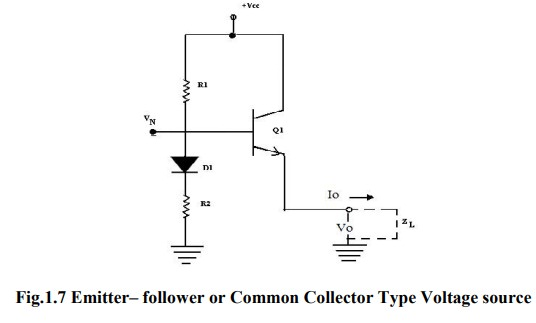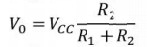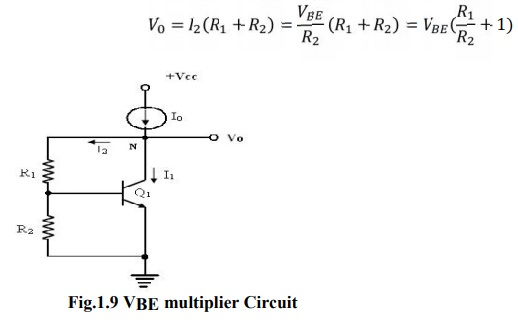Home | | Linear Integrated Circuits | Voltage Sources

# Voltage Sources

A voltage source is a circuit that produces an output voltage V0, which is independent of the load driven by the voltage source, or the output current supplied to the load.

Voltage Sources

A voltage source is a circuit that produces an output voltage V0, which is independent of the load driven by the voltage source, or the output current supplied to the load. The voltage source is the circuit dual of the constant current source.

A number of IC applications require a voltage reference point with very low ac impedance and a stable dc voltage that is not affected by power supply and temperature variations. There are two methods which can be used to produce a voltage source, namely,

1.        Using the impedance transforming properties of the transistor, which in turn determines the current gain of the transistor and

2.        Using an amplifier with negative feedback.

## Voltage source circuit using Impedance transformation:

The voltage source circuit using the impedance transforming property of the transistor is shown in figure. The source voltage Vs drives the base of the transistor through a series resistance RS and the output is taken across the emitter. From the circuit, the output ac resistance looking Into emitter is given byIt is to be noted that, equation is applicable only for small changes in the output current. The load regulation parameter indicates the changes in V0 resulting from large changes in output current I0 , Reduction in V0 occurs as I0 goes from no-load current to full-load current and this factor determines the output impedance of the voltage sources.

## Emitter– follower or Common Collector Type Voltage source:

The figure shows an emitter follower or common collector type voltage source.This voltage source is suitable for the differential gain stage used in op-amps. This circuit has the advantages of

A.      Producing low ac impedance and

B.      Resulting in effective decoupling of adjacent gain stages.

The low output impedance of the common-collector stage simulates a low impedance voltage

source with an output voltage level of V0 represented= byThe diode D1 is used for offsetting the effect of dc value VBE , across the E-B junction of the transistor, and for compensating the temperature dependence of VBE drop of Q1. The load ZL shown in dotted line represents the circuit biased by the current through Q1.

The impedance R0 looking into the emitter= of  Q1 derived from the hybrid π model is given by## Voltage Source Using Temperature compensated Avalanche Diode

The voltage source using common collector stage has the limitations of its vulnerability for changes in bias voltage VN and the output voltage V0 with respect to changes in supply voltage Vcc. This is overcome in the voltage source circuit using the breakdown voltage of the base- emitter junction shown below.

The emitter – follower stage of common – collector is eliminated in this circuit, since the impedance seen looking into the bias terminal N is very low. The current source I1 is normally simulated by a resistor connected between Vcc and node n. Then, the output voltage level V0 at node N is given by V0 = VB +VBE Where VB is the breakdown voltage of diode DB and VBE is the diode drop across D1.

The breakdown diode DB is normally realized using the base-emitter junction of the transistor. The diode D1 provides partial compensation for the positive temperature coefficient effect of VB. In a monolithic IC structure, DB and D1 can be conveniently realized as a single transistor with two individual emitters as shown in figure.## Voltage source using breakdown voltage of the base- emitter junction

The structure consists of composite connection of two transistors which are diode-connected back-to back. Since the transistors have their base to collector terminals common, they can be designed as a single transistor with two emitters.

The output resistance R0 looking into the output terminal in figure is given by Ro=RB+VT/I1 where RB and VT/I1 are the ac resistances of the base–emitter resistance of diode DB and D1 respectively. Typically RB is in the range of 40Ω to 100Ω, and V0 in the range of 6.5V to 9V.

## Voltage Source using VBE as a reference:

The output stage of op-amp requires stabilized bias voltage source, which can be obtained using a forward-biased diode connected transistor. The forward voltage drop for such a connection is approximately 0.7V, and it changes slightly with current.

When a voltage level greater than 0.7V, is needed, several diodes can be connected in series, which can offer integral multiples of 0.7V. Alternatively, the figure shows a multiplier circuit, which can offer voltage levels that need not be integral multiplied of 0.7V. The drop across R2 equals VBE drop of Q1. Considering negligible base current for Q1, current through R2 is the same as that flowing through R1.

Therefore, the output voltage V0 can be expressed asHence, the voltage V0 can be any multiple of VBE by properly selecting the resistors R1 and R2. Due to the shunt feedback provided by R1, the transistor current I1 automatically adjusts itself, towards maintaining I2 and V0 relatively independent of the changes in supply voltage.

The ac output resistance of the circuit R0 is given= by,Tags : Operational Amplifiers , Linear Integrated Circuits : Basics of Operational Amplifiers
Study Material, Lecturing Notes, Assignment, Reference, Wiki description explanation, brief detail
Linear Integrated Circuits : Basics of Operational Amplifiers : Voltage Sources | Operational Amplifiers